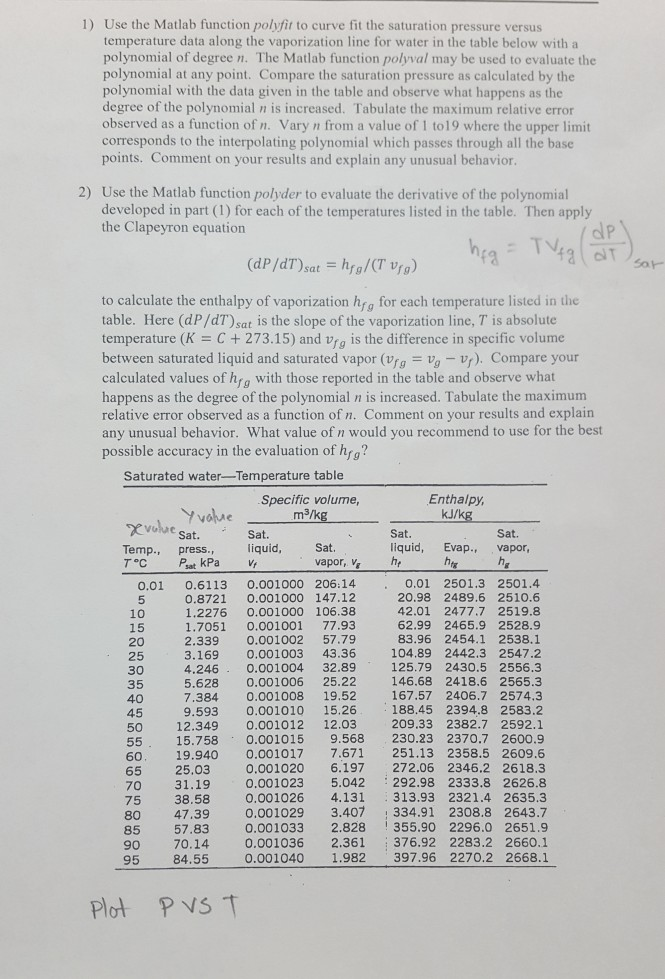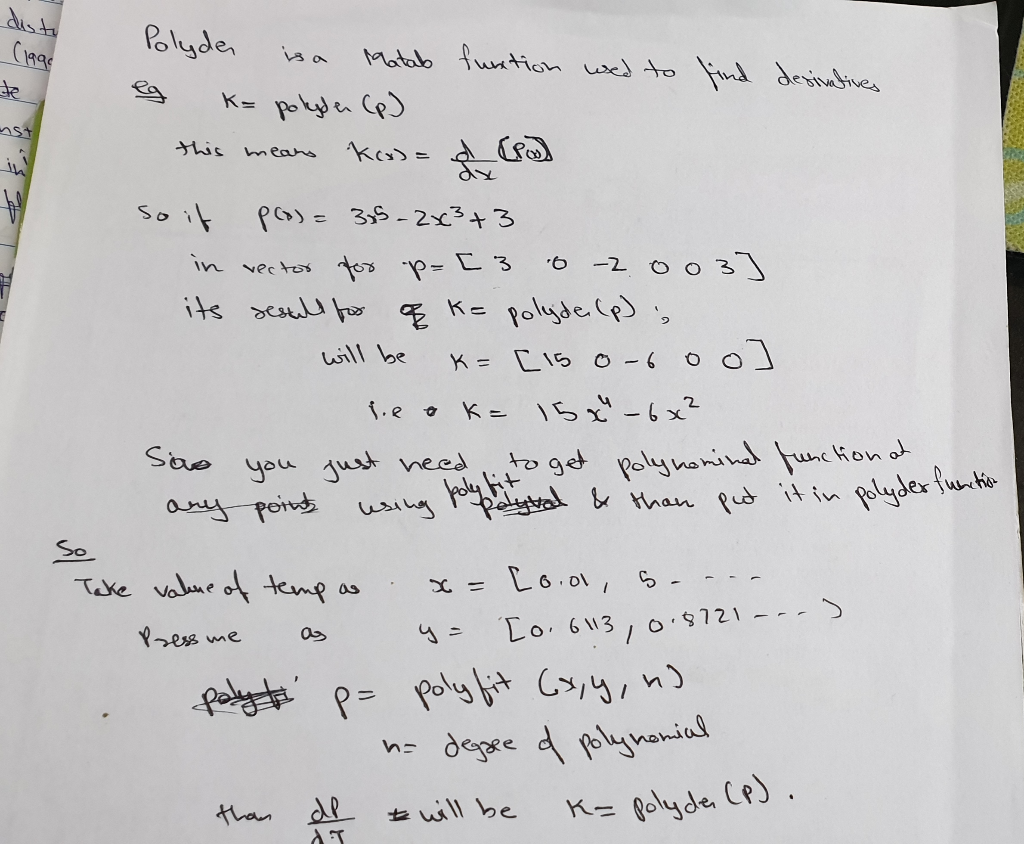# 1) Use the Matlab function polyfit to curve fit the saturation pressure versus temperature data a...MATLAB Code for question (2)

1) Use the Matlab function polyfit to curve fit the saturation pressure versus temperature data along the vaporization line for water in the table below with a polynomial of degree n. The Matlab function polyval may be used to evaluate the polynomial at any point. Compare the saturation pressure as calculated by the polynomial with the data given in the table and observe what happens as the degree of the polynomial n is increased. Tabulate the maximum relative error observed as a function of n. Vary n from a value of 1 to19 where the upper limit corresponds to the interpolating polynomial which passes through all the base points. Comment on your results and explain any unusual behavior. 2) Use the Matlab function polyder to evaluate the derivative of the polynomial developed in part (1) for each of the temperatures listed in the table. Then apply the Clapeyron equation to calculate the enthalpy of vaporization hrg for each temperature listed in the table. Here (dP/dT)sat is the slope of the vaporization line, T is absolute temperature (K C+ 273.15) and vrg is the difference in specific volume between saturated liquid and saturated vapor (Vrg V-v). Compare your calculated values of hrg with those reported in the table and observe what happens as the degree of the polynomial n is increased. Tabulate the maximum relative error observed as a function of n. Comment on your results and explain any unusual behavior. What value of n would you recommend to use for the best possible accuracy in the evaluation of hg? Saturated water-Temperature table Sat. hr 0.01 0.6113 0.001000 206:14 0.8721 0.001000 147.12 1.2276 0.001000 106.38 1.7051 0.001001 77.93 2.339 0.001002 57.79 3.169 0.001003 43.36 4.246 0.001004 32.89 5.628 0.001006 25.22 7.384 0.001008 19.52 9.593 0.001010 15.26 12.349 0.001012 12.03 0.01 2501.3 2501.4 20.98 2489.6 2510.6 42.01 2477.7 2519.8 62.99 2465.9 2528.9 83.96 2454.1 2538.1 104.89 2442.3 2547.2 125.79 2430.5 2556.3 146.68 2418.6 2565.3 167.57 2406.7 2574.3 188.45 2394.8 2583.2 209.33 2382.7 2592. 15.758 0.001015 9.568 230.23 2370.7 2600.9 19.940 0.001017 7.671 251.13 2358.5 2609.6 0.001020 6.197 272.06 2346.2 2618.3 0.001023 5.042 292.98 2333.8 2626.8 75 38.58 0.001026 4.131 313.93 2321.4 2635.3 47.39 0,001029 3.407 334.91 2308.8 2643.7 0.001033 2.828 355.90 2296.0 2651.9 0.001036 2.361 376.92 2283.2 2660.1 95 84.55 0.001040 1.982 397.96 2270.2 2668.1 Plot